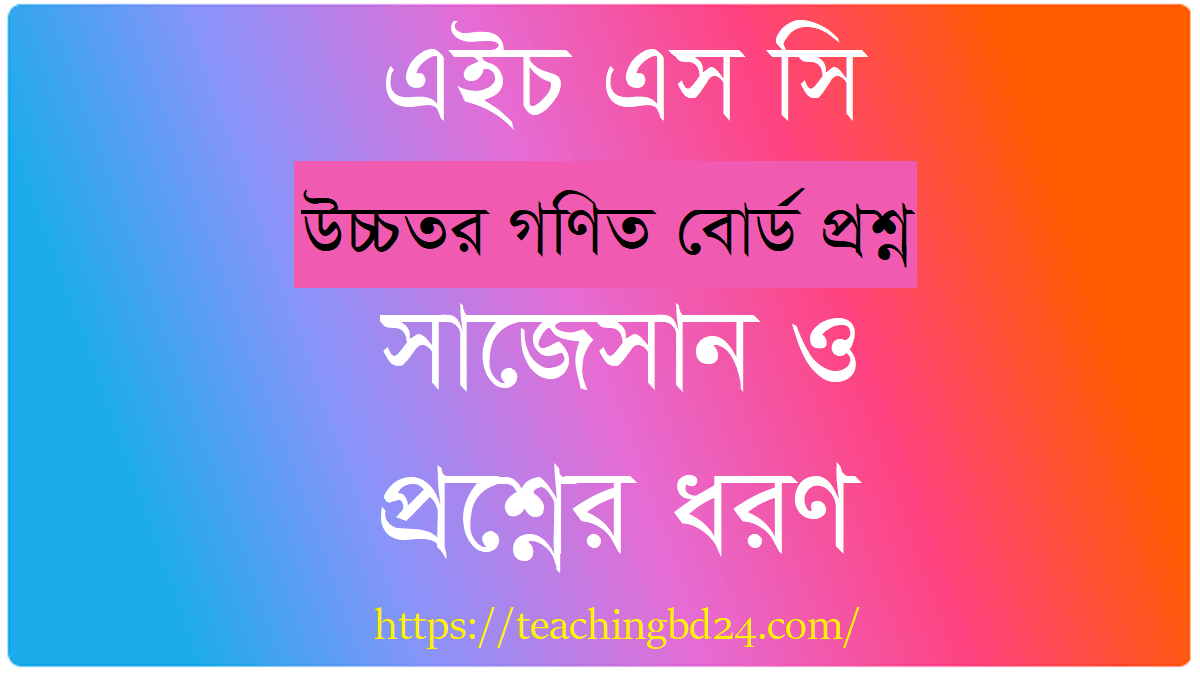# HSC All Board Higher Mathematics 2nd Paper Board Question 2018

HSC All Board Higher Mathematics 2nd Paper Board Question 2018. Mathematicians seek patterns and formulate new conjectures. Mathematicians solve the truth or falsity of conjectures by mathematical proof. The research needed to solve mathematical problems can take years, or even centuries of investigation supported. Since the pioneering work of Giuseppe Peano (1858-1932), David Hilbert (1862-1943), and others on the axiomatic systems by the end of the 19th century, it became customary to show that mathematical research establishing the truth by rigorous deduction from axioms of appropriately chosen and definitions. When these structures are good mathematical models of real phenomena, mathematical reasoning can give an overview or predictions on nature.

## HSC All Board Higher Mathematics 2nd Paper Board Question 2018# 2nd Paper

HSC Higher Mathematics 2nd Paper Question 2018 Dhaka, Dinajpur, Sylhet, Jashore Board

HSC Higher Mathematics 2nd Paper Question 2018 Rajshahi, Cumilla, Chattogram, Barishal Board

Mathematics has no generally accepted definition. Aristotle defined mathematics as “the science of quantity” and this definition prevailed until the 18th century. In the 19th century, when the study of mathematics increased in rigor and began to address abstract topics such as group theory and projective geometry, which have no clear-cut relation to quantity and measurement, mathematicians and philosophers began to propose a variety of new definitions. Three leading types of definitions of mathematics today are called logicist, intuitionist, and formalist, each reflecting a different philosophical school of thought. All have severe flaws, none has widespread acceptance, and no reconciliation seems possible.

An early definition of mathematics in terms of logic was Benjamin Peirce’s “the science that draws necessary conclusions” (1870). In the Principia Mathematica, Bertrand Russell and Alfred North Whitehead advanced the philosophical program known as logicism and attempted to prove that all mathematical concepts, statements, and principles can be defined and proved entirely in terms of symbolic logic. A logicist definition of mathematics is Russell’s “All Mathematics is Symbolic Logic” (1903).

Intuitionist definitions, developing from the philosophy of mathematician L. E. J. Brouwer, identify mathematics with certain mental phenomena. An example of an intuitionist definition is “Mathematics is the mental activity which consists in carrying out constructs one after the other.” A peculiarity of intuitionism is that it rejects some mathematical ideas considered valid according to other definitions. In particular, while other philosophies of mathematics allow objects that can be proved to exist even though they cannot be constructed, intuitionism allows only mathematical objects that one can actually construct. Intuitionists also reject the law of excluded middle — a stance that forces them to reject proof by contradiction as a viable proof method as well.

Formalist definitions identify mathematics with its symbols and the rules for operating on them. Haskell Curry defined mathematics simply as “the science of formal systems”. A formal system is a set of symbols, or tokens, and some rules on how the tokens are to be combined into formulas. In formal systems, the word axiom has a special meaning different from the ordinary meaning of “a self-evident truth”, and is used to refer to a combination of tokens that is included in a given formal system without needing to be derived using the rules of the system.

A great many professional mathematicians take no interest in a definition of mathematics or consider it undefinable. There is not even consensus on whether mathematics is an art or a science. Some just say, “Mathematics is what mathematicians do.”

teachingbd24.com is such a website where you would get all kinds of necessary information regarding educational notes, suggestions and questions’ patterns of school, college, and madrasahs. Particularly you will get here special notes of physics that will be immensely useful to both students and teachers. The builder of the website is Mr. Md. Shah Jamal Who has been serving for 30 years as an Asst. Professor of BAF Shaheen College Dhaka. He expects that this website will meet up all the needs of Bengali version learners /students. He has requested both concerned students and teachers to spread this website home and abroad.

HSC All Board All Subjects Board Question 2018

এখানে ক্লিক করে ২০১৭ সালের বিভিন্ন বিষয়ের বোর্ড প্রশ্ন দেখে নিন।

Facebook Comments